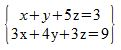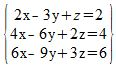# How to Solve a Linear System in Three Variables With No or Infinite Solutions

Instructions:

Choose an answer and hit 'next'. You will receive your score and answers at the end.

question 1 of 3

### How many more equations are needed to complete this linear system with three variables?Create Your Account To Take This Quiz

As a member, you'll also get unlimited access to over 75,000 lessons in math, English, science, history, and more. Plus, get practice tests, quizzes, and personalized coaching to help you succeed.

Try it risk-free for 30 days. Cancel anytime.

### 2. How many solutions does this system have?Create your account to access this entire worksheet
A Premium account gives you access to all lesson, practice exams, quizzes & worksheets
Quizzes, practice exams & worksheets
Certificate of Completion
Create an account to get started

Linear systems in three variables can have no or infinite solutions. This quiz and worksheet combination will help you test your understanding of these types of systems. These practice questions will help you test yourself on how to complete these systems and how to identify whether a system has no or infinite solutions.

## Quiz & Worksheet Goals

In these assessments, you'll be tested on:

• The characteristics of linear systems
• Amount of equations or solutions a system has
• Identifying linear systems with no solutions

## Skills Practiced

This quiz and worksheet will test your skills in the following areas:

• Reading comprehension - ensure that you draw the pertinent information on these systems from the related lesson
• Critical thinking - apply relevant concepts to examine information about linear systems in a different light
• Problem solving - use your acquired knowledge to solve linear system practice problems
• Knowledge application - use your knowledge to answer questions about linear systems with no or infinite solutions

To learn more about solving linear systems in three variables with no or infinite solutions, review the accompanying lesson How to Solve a Linear System in Three Variables With No or Infinite Solutions. This lesson covers the following objectives:

• Define linear systems in three variables
• Differentiate between systems with no and infinite solutions
• Identify the characteristics of each type of linear system
• Understand how to use the elimination method to solve these systems
Final ExamAlgebra II: High School
Status: Not Started
Chapter ExamAlgebra II: Systems of Linear Equations
Status: Not Started

Support